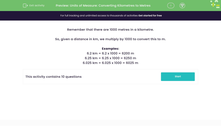# Units of Measure: Converting Kilometres to Metres

In this worksheet, students convert distances given in kilometres into metres.Key stage:  KS 2

Curriculum topic:   Measurement

Curriculum subtopic:   Convert Between Metric Measurements

Difficulty level:#### Worksheet Overview

Remember that there are 1000 metres in a kilometre.

So, given a distance in km, we multiply by 1000 to convert this to m.

Examples:

6.2 km = 6.2 x 1000 = 6200 m

6.25 km = 6.25 x 1000 = 6250 m

6.025 km = 6.025 x 1000 = 6025 m

### What is EdPlace?

We're your National Curriculum aligned online education content provider helping each child succeed in English, maths and science from year 1 to GCSE. With an EdPlace account you’ll be able to track and measure progress, helping each child achieve their best. We build confidence and attainment by personalising each child’s learning at a level that suits them.

Get started